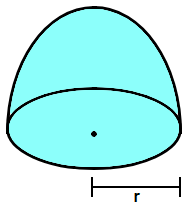Courses
Courses for Kids
Free study material
Offline Centres
MoreLast updated date: 04th Dec 2023
Total views: 279.9k
Views today: 6.79k

# What is the ratio of the T.S.A of the solid hemi – sphere to the square of its radius.Verified
279.9k+ views
Hint: Assume the required ratio of the T.S.A of the solid – hemisphere and the square of its radius as R. Assume that the radius of the solid hemisphere is r. Now, apply the formula for the Total Surface Area of the solid hemisphere given as T.S.A = $3\pi {{r}^{2}}$. Take the expression of T.S.A in the numerator and the square of the radius $\left( {{r}^{2}} \right)$ in the denominator. Cancel the common factors to get the answer.

Complete step by step solution:
Here we have been provided with a solid hemisphere and we are asked to find the ratio of the Total Surface Area of the hemisphere to the square of its radius. Let us assume the required ratio is R.Now, let us assume the radius of the hemisphere is r. We know that the Total Surface Area of a solid hemisphere is given by the formula T.S.A = $3\pi {{r}^{2}}$. The square of the radius of the hemisphere will be equal to $\left( {{r}^{2}} \right)$. According to the question we have to take the ratio of T.S.A to the square of the radius that means T.S.A will be in the numerator of the fraction while $\left( {{r}^{2}} \right)$ will be in the denominator. So, mathematically we have the ratio (R) as: -
$\Rightarrow R=\dfrac{3\pi {{r}^{2}}}{{{r}^{2}}}$
Cancelling the common factors and converting the fraction into the ratio we get,
\begin{align} & \Rightarrow R=\dfrac{3\pi }{1} \\ & \therefore R=3\pi :1 \\ \end{align}
Hence, the required ratio is $3\pi :1$.

Note: Note that we get a hemisphere when we cut a sphere into two halves. The total surface area of a sphere is $4\pi {{r}^{2}}$ so do not think that cutting the sphere into two halves makes the total surface area of the hemisphere half of that of sphere but only the volume gets halved. Remember the formulas for a solid sphere: - Curved Surface Area = $2\pi {{r}^{2}}$ and Volume = $\dfrac{2}{3}\pi {{r}^{3}}$.